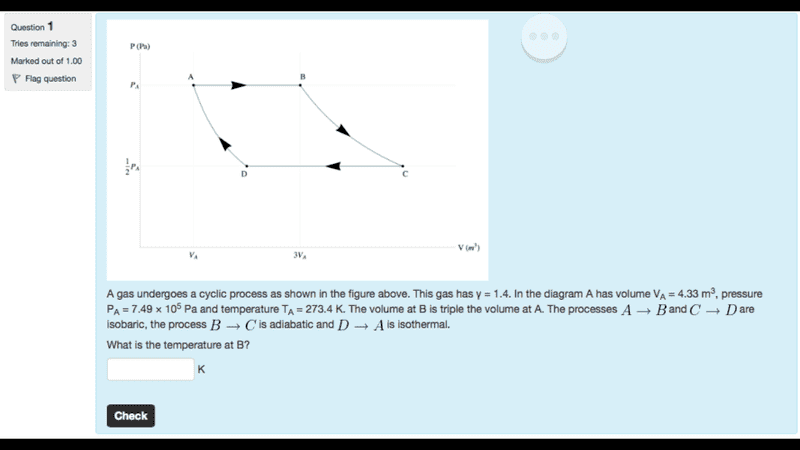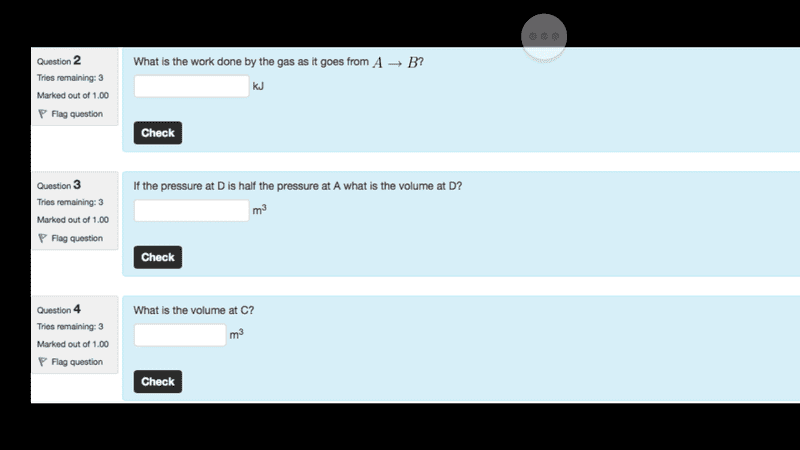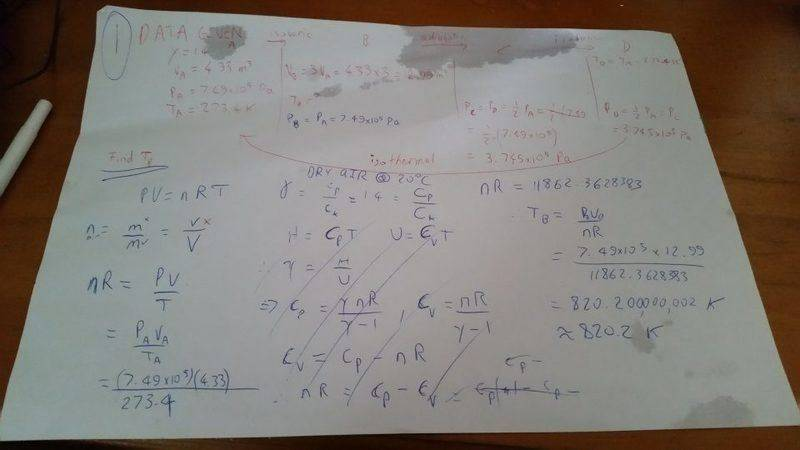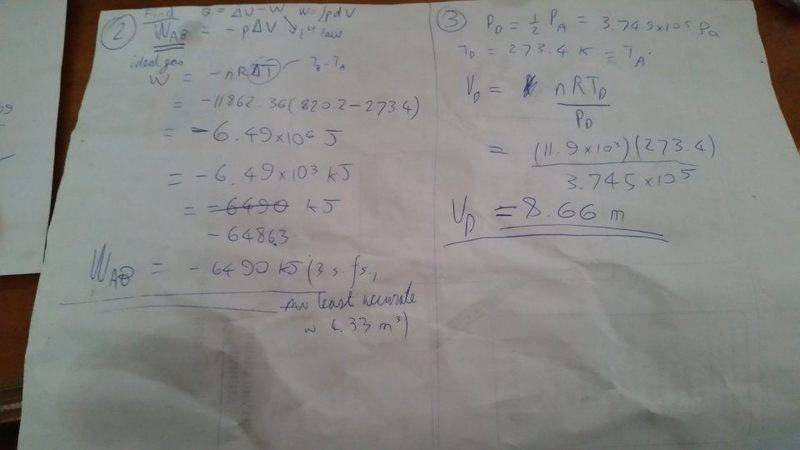# Solve Ideal Gas Problem: Gamma = 1.4, VA=4.33m^3, PA

• James Ray

## Homework Statement

A gas undergoes a cyclic process as shown in the figure above. The gas has gamma = 1.4. In the diagram A has volume V_A = 4.33 m^3, pressure P_A## Homework Equations## The Attempt at a Solution[/B]

#### Attachments

Last edited:
Your answers for Q 1,2 and 3 look correct. Have you attempted Q4?

Hint:

If I remember correctly for an adiabatic process..

P1V1γ = P2V2γ

If I've got that wrong perhaps someone can correct me.